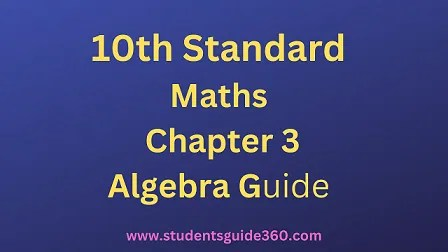# 10th Maths Chapter 3 Algebra Exercise 3.16

10th Standard Maths Chapter 3 Algebra Samacheer Kalvi Guide Exercise 3.16 Book Back Answers Solutions. TN 10th SSLC Samacheer Kalvi Guide. 10th All Subject Guide – Click Here. Class 1 to 12 All Subject Book Back Answers – Click Here# TN 10th STD Maths Chapter 3 Algebra Exercise 3.16• (i) The number of elements
• (ii) The order of the matrix
• (iii) Write the elements a22, a23, a24, a34, a43, a44

Solution:
(i) 16
(ii) 4 × 4
(iii) 7–√,3√2, 5, 0, -11, 1

### 2. If a matrix has 18 elements, what are the possible orders it can have? What if it has 6 elements?

Solution:
1 × 18, 2 × 9, 3 × 6, 6 × 3, 9 × 2, 18 × 1 and 1 × 6, 2 × 3, 3 × 2, 6 × 1

### 3. Construct a 3 × 3 matrix whose elements are given by then find the transpose of A.

(i) aij = |i – 2j|
(ii) aij = (i+j)33
Solution:
(i) aij = |i – 2j|
a11 = |1 – 2 × 1| = |1 – 2| = |-1| = 1
a12 = |1 – 2 × 2| = |1 – 4| = |-3| = 3
a13 = |1 – 2 × 3| = |1 – 6| = |-5| = 5
a21 = |2 – 2 × 1| = |2 – 2| = 0
a22 = |2 – 2 × 2| = |2 – 4| = |-2| = 2
a23 = |2 – 2 × 3| = |2 – 6| = |-4| = 4
a31 = |3 – 2 × 1| = |3 – 2| = |1| = 1
a32 = |3 – 2 × 2| = |3 – 4| = |-1| = 1
a33 = |3 – 2 × 3| = |3 – 6| = |-3| = 3
∴ ⎡⎣⎢101321543⎤⎦⎥ is the required 3 × 3 matrix4.Solution:5.Solution:6.Solution:### 7. Find the values of x,y and z from the following equationsSolution: